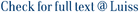We consider sparse digraphs generated by the configuration model with given in-degree and out-degree sequences. We establish that with high probability the cover time is linear up to a poly-logarithmic correction. For a large class of degree sequences we determine the exponent γ≥1 of the logarithm and show that the cover time grows as nlogγ(n), where n is the number of vertices. The results are obtained by analysing the extremal values of the stationary distribution of the digraph. In particular, we show that the stationary distribution π is uniform up to a poly-logarithmic factor, and that for a large class of degree sequences the minimal values of π have the form 1nlog1−γ(n), while the maximal values of π behave as 1nlog1−κ(n) for some other exponent κ∈[0,1]. In passing, we prove tight bounds on the diameter of the digraphs and show that the latter coincides with the typical distance between two vertices.

Stationary distribution and cover time of sparse directed configuration models / Caputo, Pietro; Quattropani, Matteo. - In: PROBABILITY THEORY AND RELATED FIELDS. - ISSN 0178-8051. - (In corso di stampa), pp. ---. [10.1007/s00440-020-00995-6]

### Stationary distribution and cover time of sparse directed configuration models

#### Abstract

We consider sparse digraphs generated by the configuration model with given in-degree and out-degree sequences. We establish that with high probability the cover time is linear up to a poly-logarithmic correction. For a large class of degree sequences we determine the exponent γ≥1 of the logarithm and show that the cover time grows as nlogγ(n), where n is the number of vertices. The results are obtained by analysing the extremal values of the stationary distribution of the digraph. In particular, we show that the stationary distribution π is uniform up to a poly-logarithmic factor, and that for a large class of degree sequences the minimal values of π have the form 1nlog1−γ(n), while the maximal values of π behave as 1nlog1−κ(n) for some other exponent κ∈[0,1]. In passing, we prove tight bounds on the diameter of the digraphs and show that the latter coincides with the typical distance between two vertices.
##### Scheda breve Scheda completa Scheda completa (DC)In corso di stampa
Stationary distribution and cover time of sparse directed configuration models / Caputo, Pietro; Quattropani, Matteo. - In: PROBABILITY THEORY AND RELATED FIELDS. - ISSN 0178-8051. - (In corso di stampa), pp. ---. [10.1007/s00440-020-00995-6]
File in questo prodotto:
File
Caputo-Quattropani2020_Article_StationaryDistributionAndCover.pdf

Open Access

Tipologia: Versione dell'editore
Licenza: Creative commons
Dimensione 781.3 kB
Utilizza questo identificativo per citare o creare un link a questo documento: `https://hdl.handle.net/11385/196435`
•4
•4